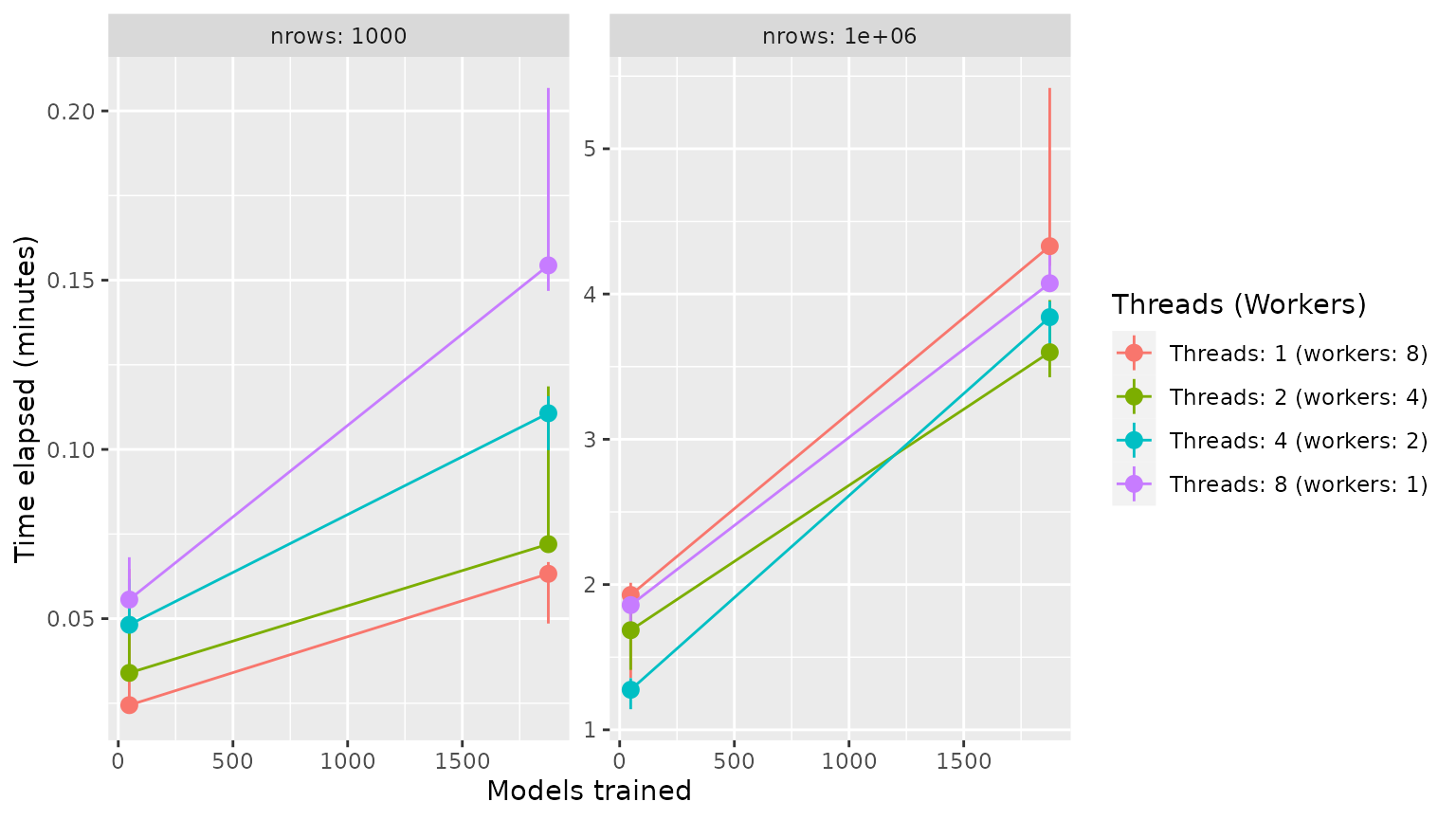## Rules of thumb

• When tuning: doParallel::registerDoParallel(8) then tune_grid()/tune_bayes()
• When fitting: set_engine("catboost", nthread = 8) then fit()/last_fit()

## Packages and data

library(tidymodels)
library(treesnip)

big_mtcars <- mtcars %>% sample_n(100000, replace = TRUE)

## Forking (workers)

Set doParallel::registerDoParallel(8) before tune_grid() or tune_bayes() and you are good to go. Just a reminder to watch out the memory usage. Forking can make your machine run out of memory.

# model spec
model <- boost_tree(tree_depth = tune()) %>%
set_mode("regression") %>%
set_engine("catboost")
# set_engine("lightgbm")

# recipe
rec <- recipe(mpg ~ ., data = big_mtcars)

# workflows

# parallel tune grid
doParallel::registerDoParallel(8)
tg <- tune_grid(wf, vfold_cv(big_mtcars, 5))

In summary, all you have to do is set nthread = x where x is the number of threads to use, usually the number of CPU cores to let the engine use from you own computer. E.g. set_engine("lightgbm", nthread = 8).

Parsnip principles states that parallel processing must be explictly requested by the user1, so if nthread were not specified, just a single thread will be used. 

model <- boost_tree(tree_depth = tune()) %>%
set_mode("regression") %>%
finalize_model(select_best(tg, "rmse"))

definitive_fit <- fit(wf,  big_mtcars)

PS: originally, there is no nthread parameter for {catboost} but {treesnip} made nthread an alias for thread_count for convenience.

## Tune Grid Benchmark (speed)

### Findings:

• Threading is better for small datasets
• Forking is better for large datasets
• Memory is an issue when forking

### Parameters (all combinations of…):

• the size of the data.frame: 1.000 vs 1.000.000 rows
• the number of models trained in grid search: 48 vs 1875
• threads/workers: 1/8, 2/4, 4/2 and 8/1 (there was a constraint of workers*threads = 8)

### Machine:

• Intel Core i7-8565U
• CPU @ 1.80GHz × 8 cores
• Dell Inspiron
• RAM 16GB
• Linux Ubuntu 20.04 64bit

### Code:

library(tidymodels)
library(treesnip)

# main function to tune_grid with given benchmark parameters
execute_tune_grid <- function(df, threads, workers, cv_folds, tune_grid, engine = "lightgbm") {
##############################################################
df_splits <- vfold_cv(df, v = cv_folds)
df_rec <- recipe(mpg ~ ., data = df)
df_model <- boost_tree(
mtry = 3,
trees = 600,
sample_size = 0.7,
min_n = tune(),
tree_depth = tune(),
learn_rate = tune(),
loss_reduction = tune()
) %>%
set_mode("regression") %>%

df_wf <- workflow() %>%

##############################################################
doParallel::registerDoParallel(workers)
tg <- tune_grid(df_wf, df_splits, grid = tune_grid)

tg
}

# parameters
parameters <- expand.grid(
engine = "lightgbm",
cv_folds = 3,
tune_grid = c(2, 5),
nrow = c(1e3, 1e6),
threads = c(1, 2, 4, 8)
) %>%
mutate(
)

# bench::mark
set.seed(1)
bm <- bench::press(
{
Sys.sleep(3)
df <- mtcars %>% sample_n(nrow, replace = TRUE)
bench::mark(
execute_tune_grid(df, threads, workers, cv_folds, tune_grid, engine),
check = FALSE,
iterations = 3,
memory = FALSE,
filter_gc = FALSE,
time_unit = "m"
)
},
.grid = parameters
)

### Results:

bm %>%
mutate(
nrow = paste("nrows:", nrow),
models_trained = (tune_grid ^ 4) * cv_folds,
max = map_dbl(time, max)/60,
labs(x = "Models trained", y = "Time elapsed (minutes)", colour = "Threads (Workers)")`